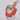# When to use call_user_func() and 'funcname'() in PHP?

PHP

There are 2 ways to call a function whose name is set at runtime in PHP. One is `call_user_func()` and another is `'funcname'()`.

``````<?php

\$funcname = 'array_sum';

// call_user_func()
call_user_func(\$funcname, [1, 2, 3]);  // => 6

// 'funcname'()
\$funcname([1, 2, 3]);  // => 6``````

At a glance these 2 methods are completely same but there are some important differences between them. Let's check them one by one.

## `call_user_func()`

• Can take anonymous functions (which is called "closure" in PHP).

• Can take instance methods.

``````<?php

class Groot {
public function talk() {
print 'I am Groot.';
}
}

// Instance methods can be passed.
\$g = new Groot();
call_user_func([\$g, 'talk']);  // => I am Groot.``````
• Can take static methods.
``````<?php

class Terminator {
public static function sayGoodbye() {
print 'Hasta la vista, baby.';
}
}

// Static methods can be passed.
call_user_func('Terminator::sayGoodbye');  // => Hasta la vista, baby.``````

## `'funcname'()`

• Can take parameters as reference. `call_user_func()` always takes parameters as value and cannot do this.
``````<?php

\$funcname = 'array_pop';

\$a = [3, 4, 5];
// Parameters can be passed as reference.
\$funcname(\$a);
var_dump(\$a);
// => array(2) {
//   =>
//   int(3)
//   =>
//   int(4)
// }``````

So, for example, if we want to call a static method, we should use `call_user_func()`. On the other hand, we can use only `\$funcname()` in order to pass parameters as reference.

These 2 methods look same but we need to know that they're completely difference 2 things.

(FYI, there's `call_user_func_array()` which takes all parameters as an array.)

Additional notes: whereas `call_user_func()` takes the parameters only as values, `call_user_func_array()` can take parameters as reference.ソフトウェア開発やマーケティング支援などをしています。